# Where Does That Variable Come From?

Most Simulink models use workspace variable to define the important parameters.  For example, setting the gain value to K and then defining K in the workspace.  I wrote about initializing these parameters in the base workspace in a recent post.  Have you ever noticed that there are many sources for these parameters?  The value of K might be in the base workspace, or it could be in the model workspace.  The source of K could even be in a mask.

Simulink determines the value of a variable by searching in the workspaces above the block that uses the variable.  Starting from the block, it searches each mask workspace up the subsystem hierarchy until it reaches the root of the model.  Simulink searches the model workspace and the base workspace last, respectively.  This process is hierarchical symbol resolution, and in this post, I will demonstrate these rules that determine how Simulink resolves the value of a variable.

An Example Model

To illustrate the concepts of hierarchical symbol resolution, let’s use a simple model (Download here).  This model consists of nothing but subsystems, constant blocks and displays.  The constant blocks refer to variables in different workspaces and get their value based on where they are in the hierarchy.  Starting at the root of the model, we find the variables k and m.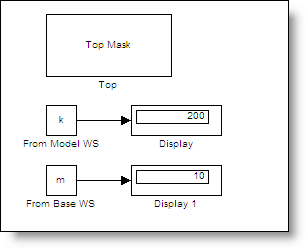Look in the Workspace above the Block

I think of the model workspace as being at the root of the model.  The model workspace is the first place to search for k and m.  I use the Model Explorer to see the contents of the model workspace.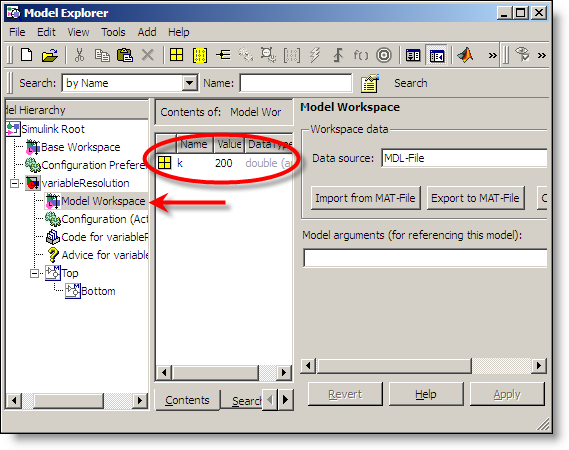We have found k, but m is not in the model workspace.  The next workspace up in the hierarchy is the base workspace.  The model explorer conveniently shows a tree that reflects the model hierarchy.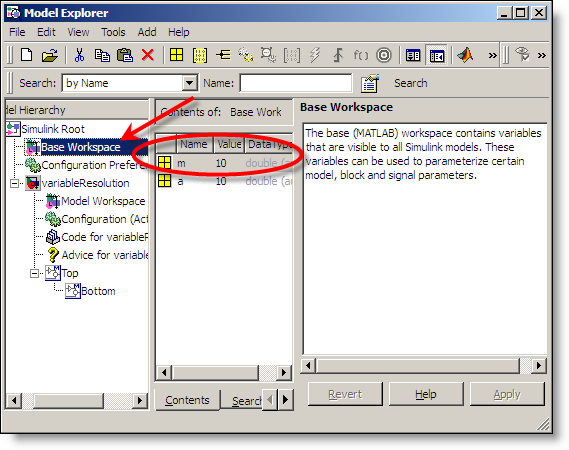Masked subsystems introduce mask workspaces in the model hierarchy.  If the block is in a masked subsystem, it first evaluates in the mask workspace then works its way up through the hierarchy until it reaches the root of the model.  Here is the Top subsystem.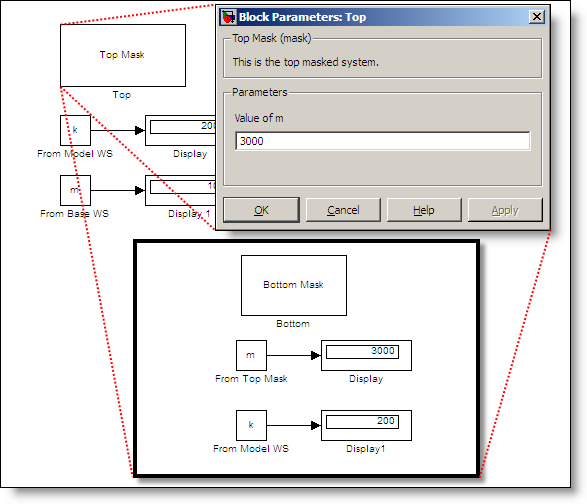The mask dialog initializes the variable m with a value of 3000.  The variable k is also appears at this level of the model, but resolves to the variable defined at the model workspace level.

Subsystems Inherit Variables from Their Context

Because of hierarchical symbol resolution, subsystems inherit variables from their context.  The Bottom subsystem refers to k, which comes from its mask.  It also refers to m, which is resolved in the Top Mask.  If the Bottom subsystem were at the root of the model, m would resolve to the base workspace.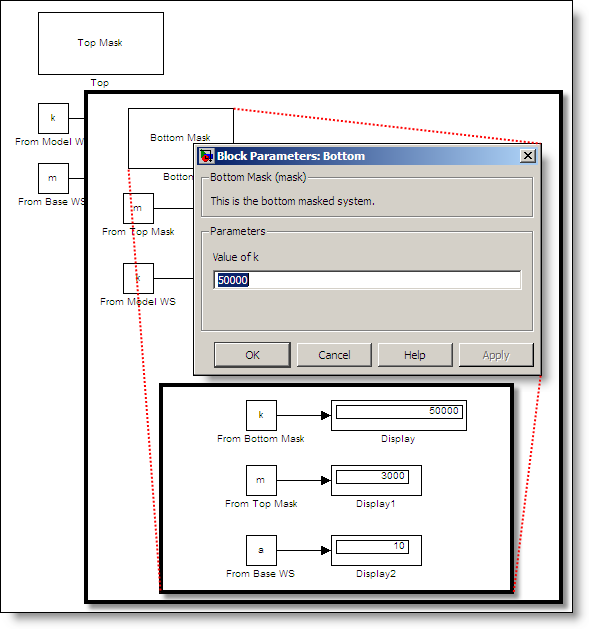This behavior can make the same library blocks behave differently depending on their parent system.  For example, a pendulum subsystem may inherit the acceleration due to gravity (g) from its parent system.  These parent system may define variables for different environments.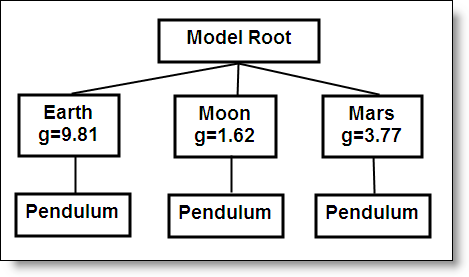Controlling Hierarchical Resolution

You can control the resolution of variables in your subsystem by setting the Permit hierarchical resolution parameter in the subsystem parameters (right click on the subsystem and select Subsystem parameters…).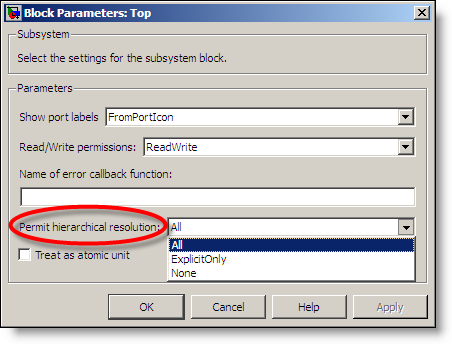The default setting is All and gives you the behavior described above.  If you don’t want variables to resolve to symbols defined in the hierarchy above your subsystem you can change this to None.   The ExplicitOnly setting controls resolution of signal and state names by only resolving objects that have been set to resolve explicitly.